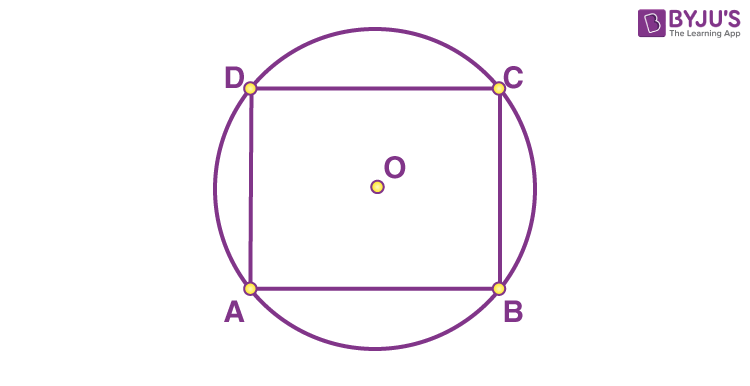# Prove that any rectangle is a cyclic quadrilateralConsider

ABCD is a rectangle

Hence,

∠A = ∠B = ∠C = ∠D = 900 [Each angle of a rectangle is 900]

⇒ ∠A + ∠C = 1800 and ∠B + ∠D = 1800

We know that,

If a pair of opposite angles of a quadrilateral is supplementary, then quadrilateral is cyclic

So, rectangle ABCD is a cyclic quadrilateral

Therefore, any rectangle is a cyclic quadrilateral(2)(0)